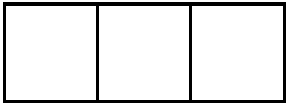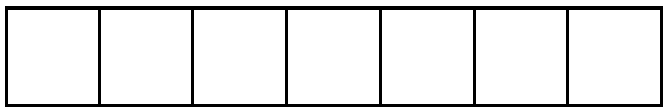# Fraction Operations

## Objective

Decompose and compose non-unit fractions greater than two as a sum of unit fractions, as a sum of non-unit fractions, and as a whole number times a unit fraction.

## Common Core Standards

### Core Standards

?

• 4.NF.B.3.B — Decompose a fraction into a sum of fractions with the same denominator in more than one way, recording each decomposition by an equation. Justify decompositions, e.g., by using a visual fraction model. Examples: 3/8 = 1/8 + 1/8 + 1/8 ; 3/8 = 1/8 + 2/8 ; 2 1/8 = 1 + 1 + 1/8 = 8/8 + 8/8 + 1/8.

• 4.NF.B.4.A — Understand a fraction a/b as a multiple of 1/b. For example, use a visual fraction model to represent 5/4 as the product 5 × (1/4), recording the conclusion by the equation 5/4 = 5 × (1/4).

?

• 3.NF.A.1

• 3.NF.A.2

## Criteria for Success

?

1. Decompose a fraction greater than 2 written as a mixed number or a fraction into a sum of unit fractions, recording the decomposition with an equation.
2. Decompose a fraction greater than 2 written as a mixed number or a fraction into a sum of fractions with the same denominator in more than one way, recording each decomposition with an equation.
3. Decompose a fraction greater than 2 written as a mixed number or a fraction into a multiple of unit fractions, recording the decomposition with an equation.
4. Compose a fraction written as a sum of unit fractions, as a sum of non-unit fractions with the same denominator, or as a multiple of a unit fraction.
5. Use decompositions of fractions as a sum of unit fractions or a multiple of a unit fraction to understand the equivalence between a mixed number and a fraction greater than 1.
6. Justify decompositions and compositions with a visual model, such as a tape diagram or number line.

## Tips for Teachers

?

#### Remote Learning Guidance

If you need to adapt or shorten this lesson for remote learning, we suggest prioritizing Anchor Task 2 (can be done independently). Find more guidance on adapting our math curriculum for remote learning here.

#### Fishtank Plus

• Problem Set
• Student Handout Editor
• Vocabulary Package

?

### Problem 1

The following image has a value of 1 whole.Using the image above, determine the value of the following shape:#### References

North Carolina Department of Public Instruction 4.NF TasksSquare Tiles

4.NF Tasks from the 3-5 Formative Instructional and Assessment Tasks for the Standards in Mathematics, made available by the North Carolina Department of Public Instruction (NCDPI) Elementary Mathematics Consultants and their public school partners under the CC BY-NC-SA 3.0 license. Accessed March 23, 2018, 1:56 p.m..

Modified by The Match Foundation, Inc.

### Problem 2

Decompose each of the following fractions as a sum of unit fractions, a sum of non-unit fractions, and a multiple of a unit fraction.

a.   ${{8\over2}}$

b.   ${5{1\over4}}$

c.   ${{12\over5}}$

### Problem 3

Compose each of the following expressions into one fraction. Write it as a fraction and a mixed number.

a.   ${{1\over3}+{1\over3}+{1\over3}+{1\over3}+{1\over3}+{1\over3}}$

b.   ${{3\over5}+{2\over5}+{6\over5}}$

c.   $12\times\frac{1}{4}$

s.   $9\times\frac{1}{2}$

## Problem Set & Homework

#### Discussion of Problem Set

• Which equations were incorrect in #1? How did you change those equations to make them correct?
• How is your work in #1(a) related to your work in #4(c)? How is adding like unit fractions related to multiplying unit fractions?
• Look at #2 and #4. Is there a way to tell when the product will be a whole number before multiplying? Explain your thinking.

?

### Problem 1

Decompose the following fractions as a sum of unit fractions and a multiple of a unit fraction.

a.   ${{10\over2}}$

b.   ${2{3\over4}}$

### Problem 2

Compose the following fractions. Write them as both a fraction greater than one and a mixed number.

a.   ${{1\over3}+{1\over3}+{1\over3}+{1\over3}+{1\over3}+{1\over3}+{1\over3}+{1\over3}+{1\over3}}$

b.   ${18\times{1\over5}}$

### Mastery Response

?